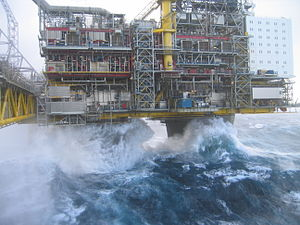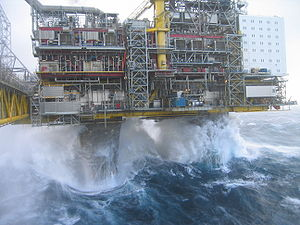# Keulegan–Carpenter number

﻿
Keulegan–Carpenter numberThe Keulegan–Carpenter number is important for the computation of the wave forces on offshore platforms.

In fluid dynamics, the Keulegan–Carpenter number, also called the period number, is a dimensionless quantity describing the relative importance of the drag forces over inertia forces for bluff objects in an oscillatory fluid flow. Or similarly, for objects that oscillate in a fluid at rest. For small Keulegan–Carpenter number inertia dominates, while for large numbers the (turbulence) drag forces are important.

The Keulegan–Carpenter number KC is defined as:$K_C = \frac{V\,T}{L},$

where:

• V is the amplitude of the flow velocity oscillation (or the amplitude of the object's velocity, in case of an oscillating object),
• T is the period of the oscillation, and
• L is a characteristic length scale of the object, for instance the diameter for a cylinder under wave loading.

A closely related parameter, also often used for sediment transport under water waves, is the displacement parameter δ:$\delta = \frac{A}{L},$

with A the excursion amplitude of fluid particles in oscillatory flow. For sinusoidal motion of the fluid, A is related to V and T as A = VT/(2π), and:$K_C = 2\pi\, \delta.\,$

The Keulegan–Carpenter number can be directly related to the Navier–Stokes equations, by looking at characteristic scales for the acceleration terms:

• convective acceleration:$(\mathbf{u}\cdot\nabla)\mathbf{u} \sim \frac{V^2}{L},$
• local acceleration:$\frac{\partial \mathbf{u}}{\partial t} \sim \frac{V}{T}.$

Dividing these two acceleration scales gives the Keulegan–Carpenter number.

A somewhat similar parameter is the Strouhal number, in form equal to the reciprocal of the Keulegan–Carpenter number. The Strouhal number gives the vortex shedding frequency resulting from placing an object in a steady flow, so it describes the flow unsteadiness as a result of an instability of the flow downstream of the object. While the Keulegan–Carpenter number is related to the oscillation frequency of an unsteady flow, into which the object is placed.

Wikimedia Foundation. 2010.

### Look at other dictionaries:

• Nusselt number — In heat transfer at a boundary (surface) within a fluid, the Nusselt number is the ratio of convective to conductive heat transfer across (normal to) the boundary. Named after Wilhelm Nusselt, it is a dimensionless number. The conductive… …   Wikipedia

• Rayleigh number — In fluid mechanics, the Rayleigh number for a fluid is a dimensionless number associated with buoyancy driven flow (also known as free convection or natural convection). When the Rayleigh number is below the critical value for that fluid, heat… …   Wikipedia

• Magnetic Reynolds number — The Magnetic Reynolds number is a dimensionless group that occurs in magnetohydrodynamics. It gives an estimate of the effects of magnetic advection to magnetic diffusion, and is typically defined by: where U is a typical velocity scale of the… …   Wikipedia

• Morton number — For Morton number in number theory, see Morton number (number theory). In fluid dynamics, the Morton number (Mo) is a dimensionless number used together with the Eötvös number to characterize the shape of bubbles or drops moving in a surrounding… …   Wikipedia

• Ohnesorge number — The Ohnesorge number, Oh, is a dimensionless number that relates the viscous forces to inertial and surface tension forces. It is defined as: Where μ is the liquid viscosity ρ is the liquid density σ is the surface tension L is the characteristic …   Wikipedia

• Deborah number — The Deborah number is a dimensionless number, often used in rheology to characterize the fluidity of materials under specific flow conditions. It was originally proposed by Markus Reiner, a professor at Technion in Israel, inspired by a verse in… …   Wikipedia

• Stanton number — The Stanton number, St or CH, is a dimensionless number that measures the ratio of heat transferred into a fluid to the thermal capacity of fluid. It is used to characterize heat transfer in forced convection flows. where h = convection heat… …   Wikipedia

• Dean number — The Dean number is a dimensionless group in fluid mechanics, which occurs in the study of flow in curved pipes and channels. It is named after the British scientist W. R. Dean, who studied such flows in the 1920s (Dean, 1927, 1928). Definition… …   Wikipedia

• Magnetic Prandtl number — The Magnetic Prandtl number is a dimensionless quantity occurring in magnetohydrodynamics which approximates the ratio of momentum diffusivity (viscosity) and magnetic diffusivity. It is defined as: where: Rem is the magnetic Reynolds number Re… …   Wikipedia

• Marangoni number — The Marangoni number (Mg) is a dimensionless number named after Italian scientist Carlo Marangoni. The Marangoni number may be regarded as proportional to (thermal ) surface tension forces divided by viscous forces. It is – for example –… …   Wikipedia